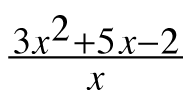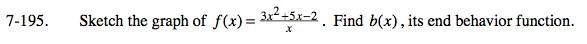### Home > CALC > Chapter 7 > Lesson 7.4.3 > Problem7-195

7-195.

Sketch the graph of. Find b(x), its end behavior function. Homework Help ✎f(x) will have a vertical asymptote at x = 0.
b(x) will not.

Divide the numerator by the denominator.
f(x) = b(x) + remainder